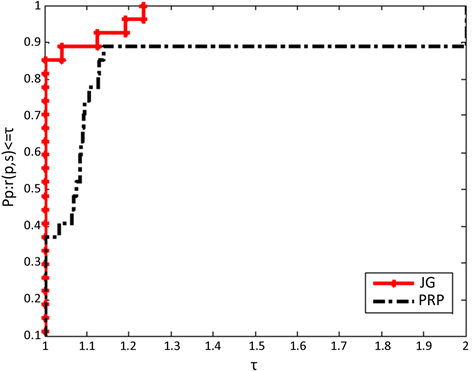# 一种新的三项共轭梯度法求解非线性方程组A New Three-Term Conjugate Gradient Method for Solving Nonlinear Equations

DOI: 10.12677/AAM.2019.85097, PDF, HTML, XML, 下载: 458  浏览: 725

Abstract: Based on the existing three-term conjugate gradient method, this paper designs a new conjugate gradient method JG to solve the problem of nonlinear equations, and proves the sufficient descent and global convergence of JG algorithm under certain assumptions. From the results of numerical experiments, we can see that JG algorithm has better properties than PRP algorithm.

1. 引言

$h\left(x\right)=0,x\in {R}^{n}$ (1)

$\mathrm{min}F\left(x\right),x\in {R}^{n}$ (2)

${x}_{k+1}={x}_{k}+{\alpha }_{k}{d}_{k}$

$\begin{array}{l}F\left({x}_{k+1}\right)-F\left({x}_{k}\right)\le {c}_{1}{\alpha }_{k}{g}_{k}^{\text{T}}{d}_{k}\\ {g}_{k+1}^{\text{T}}{d}_{k}\ge {c}_{2}{g}_{k}^{\text{T}}{d}_{k}\end{array}$

$-g{\left({x}_{k}+{\alpha }_{k}{d}_{k}\right)}^{\text{T}}{d}_{k}\ge \sigma {\alpha }_{k}‖g\left({x}_{k}+{\alpha }_{k}{d}_{k}\right)‖{‖{d}_{k}‖}^{2}$ (3)

Zhang  提出了一种三项共轭梯度算法：

${d}_{k}=\left\{\begin{array}{l}-{g}_{k}+{\beta }_{k}^{PRP}{d}_{k-1}-{v}_{k}{y}_{k}\text{}\text{ }\text{ }\text{if}\text{\hspace{0.17em}}k\ge 1\\ -{g}_{k}\text{if}\text{\hspace{0.17em}}k=0\end{array}$

${d}_{k}^{\text{T}}{g}_{k}=-{‖{g}_{k}‖}^{2}$

${d}_{k}=\left\{\begin{array}{l}-{g}_{k}+\frac{{g}_{k}^{\text{T}}{y}_{k}^{*}{d}_{k-1}-{g}_{k}^{\text{T}}{d}_{k-1}{y}_{k}^{*}}{\mu ‖{d}_{k-1}‖‖{y}_{k}^{*}‖+v{‖{y}_{k}^{*}‖}^{2}+{‖{g}_{k-1}‖}^{2}+\eta ‖{g}_{k-1}‖‖{d}_{k-1}‖+r{‖{d}_{k-1}‖}^{2}}\text{if}\text{\hspace{0.17em}}k\ge 1\\ -{g}_{k}\text{}\text{\hspace{0.17em}}\text{\hspace{0.17em}}\text{\hspace{0.17em}}\text{\hspace{0.17em}}\text{}\text{\hspace{0.17em}}\text{}\text{ }\text{ }\text{if}\text{\hspace{0.17em}}k=0\end{array}$ (4)

2. 算法

Step 0：令初始点 ${x}_{0}\in R$$\mu >0$$v>0$$\eta >0$$r>0$$\rho ,\epsilon \in \left(0,1\right)$$k:=1$

Step 1：若 $‖g\left(x\right)‖\le \epsilon$ ，停止；否则转到Step 2；

Step 2：通过(4)式计算搜索方向 ${d}_{k}$

Step 3：选择满足条件(3)的步长 ${\alpha }_{k}$

Step 4：令迭代公式为 ${w}_{k}={x}_{k}+{\alpha }_{k}{d}_{k}$

Step 5：若 $‖g\left(x\right)‖\le \epsilon$ ，停止，令 ${x}_{k+1}={w}_{k}$ ；否则令

${x}_{k+1}={x}_{k}-\frac{g{\left({w}_{k}\right)}^{\text{T}}\left({x}_{k}-{w}_{k}\right)}{{‖g\left({w}_{k}\right)‖}^{2}}g\left({w}_{k}\right)$ (5)

Step 6：令 $k:=k+1$ ，转Step 1。

3. 充分下降性和全局收敛性

$‖g\left(x\right)-g\left(y\right)‖\le L‖x-y‖,\text{}\forall x,y\in {R}^{n}$ (6)

$‖{g}_{k}‖\le \varsigma$

${g}_{k+1}{d}_{k+1}\le -{‖{g}_{k+1}‖}^{2}$ (7)

$‖{g}_{k}‖\le ‖{d}_{k}‖\le \left(1+\frac{2}{\mu }\right)‖{g}_{k}‖$ (8)

$\begin{array}{c}‖{d}_{k}‖=‖-{g}_{k}+\frac{{g}_{k}^{\text{T}}{y}_{k}^{*}{d}_{k-1}-{g}_{k}^{\text{T}}{d}_{k-1}{y}_{k}^{*}}{\mu ‖{d}_{k-1}‖‖{y}_{k}^{*}‖+v‖{y}_{k}^{*}‖+{‖{g}_{k-1}‖}^{2}+\eta ‖{g}_{k-1}‖‖{d}_{k-1}‖+r{‖{d}_{k-1}‖}^{2}}‖\\ \le ‖-{g}_{k}‖+‖\frac{{g}_{k}^{\text{T}}{y}_{k}^{*}{d}_{k-1}-{g}_{k}^{\text{T}}{d}_{k-1}{y}_{k}^{*}}{\mu ‖{d}_{k-1}‖‖{y}_{k}^{*}‖+v‖{y}_{k}^{*}‖+{‖{g}_{k-1}‖}^{2}+\eta ‖{g}_{k-1}‖‖{d}_{k-1}‖+r{‖{d}_{k-1}‖}^{2}}‖\\ \le ‖-{g}_{k}‖+\frac{2‖{g}_{k}^{\text{T}}‖‖{y}_{k}^{*}‖‖{d}_{k-1}‖}{\mu ‖{d}_{k-1}‖‖{y}_{k}^{*}‖}=\left(1+\frac{2}{\mu }\right)‖{g}_{k}‖\end{array}$

${\alpha }_{k}\ge \mathrm{min}\left\{s,\frac{\rho }{L+\sigma ‖g\left({\stackrel{^}{w}}_{k}\right)‖}\frac{{‖g\left({x}_{k}\right)‖}^{2}}{{‖{d}_{k}‖}^{2}}\right\}$

$-g{\left({x}_{k}+{\alpha }_{k}{d}_{k}\right)}^{\text{T}}{d}_{k}<\sigma {\alpha }_{k}‖g\left({x}_{k}+{\alpha }_{k}{d}_{k}\right)‖{‖{d}_{k}‖}^{2}$

${‖{g}_{k}‖}^{2}\le -{g}_{k}^{\text{T}}{d}_{k}\le {\left(g\left({\stackrel{^}{w}}_{k}\right)-g\left({x}_{k}\right)\right)}^{\text{T}}{d}_{k}+\sigma {\stackrel{^}{\alpha }}_{k}‖g\left({\stackrel{^}{w}}_{k}\right)‖{‖{d}_{k}‖}^{2}\le {\stackrel{^}{\alpha }}_{k}\left(L+\sigma ‖g\left({\stackrel{^}{w}}_{k}\right)‖\right){‖{d}_{k}‖}^{2}$

${\alpha }_{k}\ge \frac{\rho }{L+\sigma ‖g\left({\stackrel{^}{w}}_{k}\right)‖}\frac{{‖g\left({x}_{k}\right)‖}^{2}}{{‖{d}_{k}‖}^{2}}$

${‖{x}_{k+1}-{x}_{*}‖}^{2}\le {‖{x}_{k}-{x}_{*}‖}^{2}-{‖{x}_{k+1}-{x}_{k}‖}^{2}$ (9)

$\underset{k=0}{\overset{\infty }{\sum }}{‖{x}_{k+1}-{x}_{k}‖}^{2}<\infty$ (10)

$〈g\left({w}_{k}\right)-g\left({x}_{*}\right),{x}_{*}-{w}_{k}〉=〈g\left({w}_{k}\right),{x}_{*}-{w}_{k}〉\le 0$

${x}_{k+1}$${x}_{k}$${M}_{k}=\left\{x\in {R}^{n}|〈g\left({w}_{k}\right),x-{w}_{k}〉\le 0\right\}$ 上的投影。如果 ${x}_{*}$${M}_{k}$ 上，则 $〈{x}_{k}-{x}_{k+1},{x}_{k+1}-{x}_{*}〉\ge 0$ 。可得：

${‖{x}_{k}-{x}_{*}‖}^{2}={‖{x}_{k}-{x}_{k+1}‖}^{2}+{‖{x}_{k+1}-{x}_{*}‖}^{2}+2〈{x}_{k}-{x}_{k+1},{x}_{k+1}-{x}_{*}〉\ge {‖{x}_{k}-{x}_{k+1}‖}^{2}+{‖{x}_{k+1}-{x}_{*}‖}^{2}$

${‖{x}_{k+1}-{x}_{k}‖}^{2}\le {‖{x}_{k}-{x}_{*}‖}^{2}-{‖{x}_{k+1}-{x}_{*}‖}^{2}$

$‖{x}_{k+1}-{x}_{k}‖=\frac{|g{\left({w}_{k}\right)}^{\text{T}}\left({x}_{k}-{w}_{k}\right)|}{‖g\left({w}_{k}\right)‖}=\frac{-{\alpha }_{k}g{\left({w}_{k}\right)}^{\text{T}}{d}_{k}}{‖g\left({w}_{k}\right)‖}\ge \sigma {\alpha }_{k}^{2}{‖{d}_{k}‖}^{2}$

$‖{x}_{k+1}-{x}_{k}‖\to 0$

$\underset{k\to +\infty }{\mathrm{lim}}{\alpha }_{k}{d}_{k}=0$ (11)

$\underset{k\to \infty }{\mathrm{lim}}\mathrm{inf}‖{g}_{k}‖=0$ (12)

${c}_{3}\delta \le {c}_{3}‖{g}_{k}‖\le ‖{d}_{k}‖\le {c}_{4}‖{g}_{k}‖\le {c}_{4}\zeta$

$\begin{array}{c}{\alpha }_{k}‖{d}_{k}‖\ge \mathrm{min}\left\{s,\frac{\rho }{L+\sigma ‖g\left({\stackrel{^}{w}}_{k}\right)‖}\frac{{‖g\left({x}_{k}\right)‖}^{2}}{{‖{d}_{k}‖}^{2}}\right\}‖{d}_{k}‖\\ \ge \mathrm{min}\left\{{c}_{3}\delta s,\frac{\rho \delta }{{c}_{4}\zeta \left(L+\sigma \varsigma \right)}\right\}>0\end{array}$

4. 数值实验Figure 1. Algorithm JG and algorithm PRP performance chart (CT)

5. 结论

  Polyak, B.T. (1969) The Conjugate Gradient Method in Extremal Problems. Ussr Computational Mathematics & Mathematical Physics, 9, 94-112. https://doi.org/10.1016/0041-5553(69)90035-4  Dai, Y. and Yuan, Y. (1999) A Nonlinear Conjugate Gradient Method with a Strong Global Convergence Property. Siam Journal on Optimization, 10, 177-182. https://doi.org/10.1137/S1052623497318992  Fletcher, R. and Reeves, C.M. (1964) Function Minimization by Conjugate Gradients. Computer Journal, 7, 149-154. https://doi.org/10.1093/comjnl/7.2.149  Polak, E. and Ribiere, G. (1968) Note sur la convergence de methodes de directions conjuguees. Revue Française D’-informatique et de Recherche Opérationnelle, 16, 35-43. https://doi.org/10.1051/m2an/196903R100351  Wei, Z., Yao, S. and Liu, L. (2006) The Convergence Prop-erties of Some New Conjugate Gradient Methods. Applied Mathematics & Computation, 183, 1341-1350. https://doi.org/10.1016/j.amc.2006.05.150  Yuan, G. and Lu, X. (2008) A New Backtracking Inexact BFGS Method for Symmetric Nonlinear Equations. Computers & Mathematics with Applications, 55, 116-129. https://doi.org/10.1016/j.camwa.2006.12.081  Zhang, L., Zhou, W. and Li, D.H. (2006) A Descent Modified Polak-Ribiere-Polyak Conjugate Gradient Method and Its Global Convergence. IMA Journal of Numerical Analysis, 26, 629-640. https://doi.org/10.1093/imanum/drl016  Solodov, M.V. and Svaiter, B.F. (1998) A Globally Con-vergent Inexact Newton Method for Systems of Monotone Equations. Reformulation: Nonsmooth, Piecewise Smooth, Semismooth and Smoothing Methods. Springer, US, 355-369. https://doi.org/10.1007/978-1-4757-6388-1_18  Yuan, G., Wei, Z. and Lu, X. (2011) A BFGS Trust-Region Method for Nonlinear Equations. Computing, 92, 317-333. https://doi.org/10.1007/s00607-011-0146-z